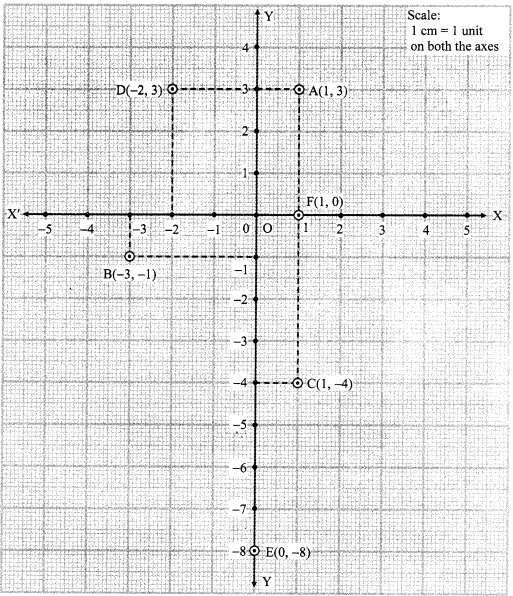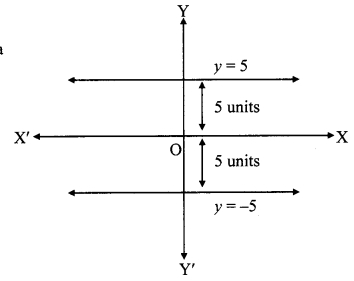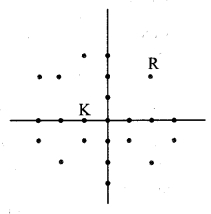# Maharashtra Board Class 9 Maths Solutions Chapter 7 Co-ordinate Geometry Problem Set 7

## Maharashtra State Board Class 9 Maths Solutions Chapter 7 Co-ordinate Geometry Problem Set 7

Question 1.
Choose the correct alternative answer for the following questions.

i. What is the form of co-ordinates of a point on the X-axis?
(A) (b,b)
(B) (0, b)
(C) (a, 0)
(D) (a, a)
(C) (a, 0)

ii. Any point on the line y = x is of the form _____.
(A) (a, a)
(B) (0, a)
(C) (a, 0)
(D) (a, -a)
(A) (a, a)

iii. What is the equation of the X-axis ?
(A) x = 0
(B) y = 0
(C) x + y = 0
(D) x = y
(B) y = 0

iv. In which quadrant does the point (-4, -3) lie ?
(A) First
(B) Second
(C) Third
(D) Fourth
(C) Third

v. What is the nature of the line which includes the points (-5, 5), (6, 5), (-3, 5), (0, 5)?
(A) Passes through the origin
(B) Parallel to Y-axis
(C) Parallel to X-axis
(D) None of these
The y co-ordinate of all the points is the same.
∴ The line which passes through the given points is parallel to X-axis.
(C) Parallel to X-axis

vi. Which of the points P(-1, 1), Q(3, -4), R( -1, -1), S(-2, -3), T (-4, 4) lie in the fourth quadrant?
(A) P and T
(B) Q and R
(C) only S
(D) P and R
(B) Q and R

Question 2.
Some points are shown in the adjoining figure. With the help of it answer the following questions :i. Write the co-ordinates of the points Q and R.
ii. Write the co-ordinates of the points T and M.
iii. Which point lies in the third quadrant ?
iv. Which are the points whose x and y co-ordinates are equal ?
Solution:
i. Q(-2, 2) and R(4, -1)
ii. T(0, -1) and M(3, 0)
iii. Point S lies in the third quadrant.
iv. The x and y co-ordinates of point O are equal.

Question 3.
Without plotting the points on a graph, state in which quadrant or on which axis do the following points lie.
i. (5, -3)
ii. (-7, -12)
iii. (-23, 4)
iv. (-9, 5)
v. (0, -3)
vi. (-6, 0)
Solution:Question 4.
Plot the following points on one and the same co-ordinate system.
A(1, 3), B(-3, -1), C(1, -4), D(-2, 3), E(0, -8), F(1, 0)
Solution:Question 5.
In the graph alongside, line LM is parallel to the Y-axis.i. What is the distance of line LM from the Y-axis?
ii. Write the co-ordinates of the points P, Q and R.
iii. What is the difference between the x co-ordinates of the points L and M?
Solution:
i. Distance of line LM from the Y-axis is 3 units.
ii. P(3, 2), Q (3, -1), R(3, 0)
iii. x co-ordinate of point L = 3
x co-ordinate of point M = 3
∴ Difference between the x co-ordinates of the points L and M = 3 – 3
= 0

Question 6.
How many lines are there which are parallel to X-axis and having a distance 5 units?
Solution:
The equation of a line parallel to the X-axis is y = b.
There are 2 lines which are parallel to X-axis and at a distance of 5 units.
Their equations are y = 5 and y = -5.Question 7.
If ‘a’ is a real number, what is the distance between the Y-axis and the line x = a?
Solution:
Equation of Y-axis is x = 0.
Since, ‘a’ is a real number, there are two possibilities.
Case I: a > 0
Case II: a < 0 ∴ Distance between the Y-axis and the line x = a = a-0 = a Since, |a| = a, a > 0
= – a, a < 0
∴ Distance between the Y-axis and the line x = a is |a|.

Maharashtra Board Class 9 Maths Chapter 7 Co-ordinate Geometry Problem Set 7 Intext Questions and Activities

Question 1.
As shown in the adjoining figure, ask girls to sit in lines so as to form the X-axis and Y-axis.
i. Ask some boys to sit at the positions marked by the coloured dots in the four quadrants.
i. Now, call the students turn by turn using the initial letter of each student’s name. As his or her initial is called, the student stands and gives his or her own co-ordinates. For example Rajendra (2, 2) and Kirti (-1, 0)
iii. Even as they have fun during this field activity, the students will leam how to state the position of a point in a plane. (Textbook pg. no. 92)